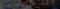Phone:(+86) 18625862121
Email:order@zzsteels.comYou are here: » » » Calculation Of Butt Welding Pipe Cross Weight

# Calculation Of Butt Welding Pipe Cross Weight

Views: 112     Author: Site Editor     Publish Time: 2023-01-31      Origin: Site

## What is a BW cross pipe?

A cross is also called four-way fitting that used to make three 90 degree branches from the main run pipeline.Depending on the way it connect，they can separated into butt welding（BW）, socket welding(SW) and threaded cross(TRD).Among them

BW cross is frequently used.Usually we can know their size and thickness by the Specification.But when we do import and export business, we need their weight calculation to decide the shipping way and do custom clearance. To help you resolve this problem, today we would like to share the BW cross weight calculator with you.

## What do we need before calculation ?

Before you do the calculation ,you need to get some data from the data sheet of BW Cross.Where OD is the specified outside diameter, expressed in millimeters (inches),T is the specified wall thickness, expressed in millimeters (inches).The data

sheet is attached.## The formula of cross weight calculation

After knowing the Nominal size and thickness of the cross,you can use the formula to do the calculation.(N is0.02466 for calculations in SI units and10.69 for calculations in USC units.)

W=N*T(OD-T)*<2(C+M)-2OD>/1000(equal cross)

W=<N*T1(OD1-T1)*(2C-OD12)+N*T2(OD2-T2)*(2M-OD1)>/1000(reducing)

Let’s take a 3”sch30 BW cross as example,is OD is 88.9(mm),the sickness is 4.78(mm),the weight is 0.02466*4.78*(88.9-4.78)*<2*(86+86)-2*88.9>/100≈1.64kg

But please note that this is the estimated weight,and the formula is based on the formula of steel pipe.The formula of BW cross is difficult to find because we always use the weight of SW and THD cross to do the calculation.

If you want to know the exact weight calculation,the best way is to weigh one sample then times the amount.